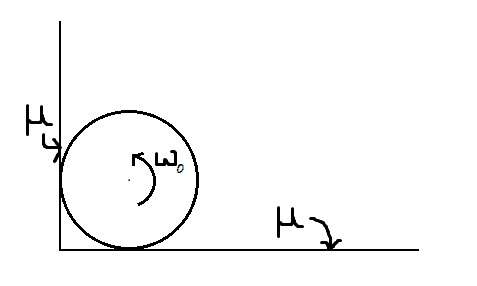# The Disc is retarded to 0 rad/sConsider a disc of mass $m$ and radius $R$ touching a rough wall of coefficient of friction $\mu$ and a rough floor of coefficient of friction $\mu$ as shown in figure. The ring has an initial angular velocity of $\omega_0$.

Find the time taken by the ring to come to a halt.

Details and Assumptions

• The disc neither translates on the floor nor on the wall

• $\mu = \dfrac{1}{2}$

• $m = 1 kg$

• $R = 4 m$

• $g = 10 m/s^2$

• $\omega_0 = 9 rad/s$

×